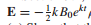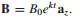### Create an Account

Already have account?

### Forgot Your Password ?

Home / Questions / In Section 91 Faradays law was used to show that the field results from the changing magne...

# In Section 91 Faradays law was used to show that the field results from the changing magnetic field a Show that these fields do not satisfy Maxwells other curl equation b If we let

In Section 9.1, Faraday’s law was used to show that the fieldresults from the changing magnetic field(a) Show that these fields do not satisfy Maxwell’s other curl equation. (b) If we let B0 = 1 T and k = 106 s−1, we are establishing a fairly large magnetic flux density in 1µs. Use the ∇ × H equation to show that the rate at which Bz should (but does not) change with ρ is only about 5 × 10−6 T per meter in free space at t = 0.

Jun 14 2020 View more View Less

#### Answer (Solved)Subscribe To Get Solution